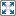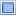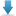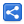# Java 9 StackWalker

[Last Updated: Apr 24, 2018]

Java 9 introduces an efficient API for obtaining stack trace information. It allows easy filtering and lazy access to the execution stacks (also called stack frames) within any method.

There are three new relevant classes we need to get familiar with:

## StackWalker

The class `java.lang.StackWalker`, is the main class we are going to work with. With this class we can do following things:

1. Traversing the `StackFrames` by using `StackWalker.forEach()` method.
2. Walk through the stack traces and applying a function (filtering and mapping etc) on a `Stream` of StackFrames by using `StackWalker.walk()` method.
3. Getting the caller class in an efficient way by calling `StackWalker.getCallerClass()`

## StackWalker.StackFrame

This class is static nested class of `StackWalker`. It represents a method invocation return by `StackWalker`. It has methods to access a given stack frame information i.e. `getDeclaringClass()`, `getLineNumber()` etc (equivalent to what we have in `StackTraceElement` class).

## StackWalker.Option

This is also a static nested class of `StackWalker`. It provides options for Stack walker to configure the stack frame information when we create the instance via `SackWalker.getInstance()`.

## Example

### Using StackWalker.forEach()

In following example, we will see how to traverse the StackFrames.

```public class StackWalkerTraversalExample {
public static void main(String[] args) {
method1();
}

private static void method1() {
method2();
}

private static void method2() {
method3();
}

private static void method3() {
StackWalker stackWalker = StackWalker.getInstance(StackWalker.Option.RETAIN_CLASS_REFERENCE);
stackWalker.forEach(StackWalkerTraversalExample::print);
}

private static void print(StackWalker.StackFrame stackFrame) {
Class<?> c = stackFrame.getDeclaringClass();
int lineNumber = stackFrame.getLineNumber();
String methodName = stackFrame.getMethodName();
System.out.printf("Class: %s, Method: %-7s, Line: %s%n",
c.getSimpleName(), methodName, lineNumber);
}
}```

#### StackWalker.Option.RETAIN_CLASS_REFERENCE

In above example, we used `StackWalker.Option.RETAIN_CLASS_REFERENCE` option which asks StackWalker to retains Class object in StackFrames. This option is necessary if we are going to use `StackFrame.getDeclaringClass()` method.

#### Output

`Class: StackWalkerTraversalExample, Method: method3, Line: 18Class: StackWalkerTraversalExample, Method: method2, Line: 13Class: StackWalkerTraversalExample, Method: method1, Line: 9Class: StackWalkerTraversalExample, Method: main   , Line: 5`

#### Lazy initialization

`StackWalker.forEach()` and `StackWalker.walk()` (next example) initialize stack frames lazily as opposed to `Thread.getStackTrace()` which eagerly captures the entire stacks. `StackWalker` class is a way to avoid the cost of loading all frames in the situations where we are only interested in the few frames on the stack.

### Using StackWalker.walk()

This method accepts a user defined `Function` having parameter of StackFrame's Stream object (supplied by the StackWalker). The implementation of this function has full power of Java 8 streams to filter, map or perform whatever operation of streams on the StackFrames. This method returns the result of the stream.

```public class StackWalkerWalkExample {
public static void main(String[] args) {
method1();
}

private static void method1() {
method2();
}

private static void method2() {
method3();
}

private static void method3() {
StackWalker stackWalker = StackWalker.getInstance();
List<String> lines = stackWalker.walk(StackWalkerWalkExample::fetchLines);
System.out.println(lines);
}

private static List<String> fetchLines(Stream<StackWalker.StackFrame> stream) {
List<String> lines = stream.filter(stackFrame -> stackFrame.getMethodName()
.startsWith("method"))
.map(stackFrame -> stackFrame.getMethodName() +
":" + stackFrame.getLineNumber())
.collect(Collectors.toList());
return lines;
}
}```

#### Output

`[method3:22, method2:17, method1:13]`

In next tutorials, we will see examples on `StackWalker.getCallerClass()` and using different Options with `StackWalk.getInstance()` method.

## Example Project

Dependencies and Technologies Used:

• JDK 9
 Java 9 StackWalker ExamplesSelect AllDownload• stack-walker-walk-examples
• src
• com
• logicbig
• example
• StackWalkerWalkExample.java

 Share